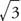## Functions of General Angles

Acute angles in standard position are all in the first quadrant, and all of their trigonometric functions exist and are positive in value. This is not necessarily true of angles in general. Some of the six trigonometric functions of quadrantal angles are undefined, and some of the six trigonometric functions have negative values, depending on the size of the angle. Angles in standard position have their terminal side in or between one of the four quadrants. Figure shows a point A (x, y) located on the terminal side of angle θ with r as the distance AO. Note that r is always positive. Based on the figures,Figure 1One way to remember which functions are positive and which are negative in the various quadrants is to remember a simple four-letter acronym, ASTC. This acronym can remind you that All are positive in quadrant I, the Sine is positive in quadrant II, the Tangent is positive in quadrant III, and the Cosine is positive in quadrant IV. This acronym could stand for Arizona State Teacher's College, AllStudents Take Classes, or some other four-word expression that will help you remember the relationships.

Table 2 summarizes the values of the trigonometric functions of quadrantal angles. Note that undefined values result from division by 0.Example 1: Find the six trigonometric functions of an angle α that is in standard position and whose terminal side passes through the point (−5, 12).Because sin θ is positive and cos θ is negative, θ must be in the second quadrant. From the Pythagorean theorem,and then it follows thatExample 3: What is the exact sine, cosine, and tangent of 330°?

Because 330° is in the fourth quadrant, sin 330° and tan 330° are negative and cos 330° is positive. The reference angle is 30°. Using the 30° − 60° − 90° triangle relationship, the ratios of the three sides are 1, 2,Therefore,